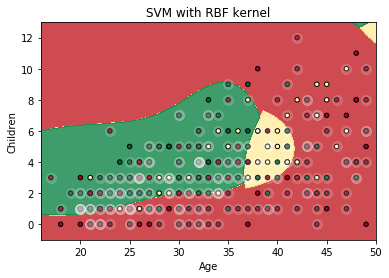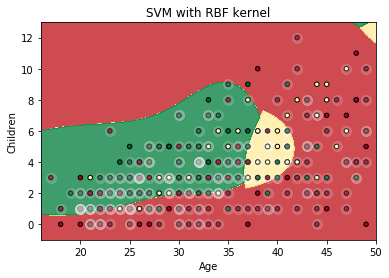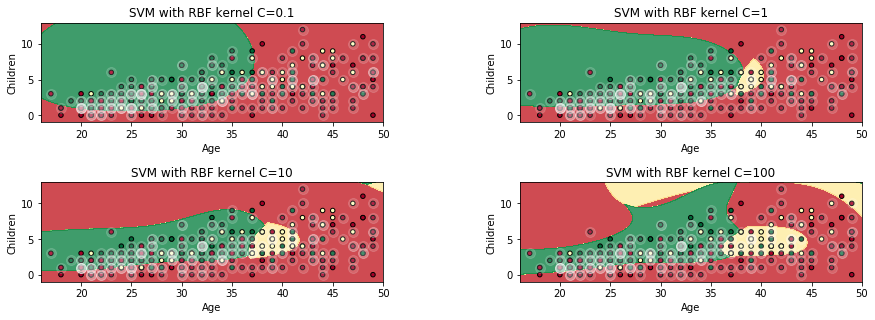# Support Vector Machines (SVM) in PythonSupport Vector Machine (SVM) is a widely used supervised learning algorithm for classification and regression tasks. It is mostly exploited for classification problems. The points of different classes are separated by a hyperplane, and this hyperplane must be chosen in such a way that the distances from it to the nearest data points on each side should be maximal. Support Vector Machine has some advantages. The first one is that SVM works well when you have highly dimensional space, for example, in text classification. Another SVM advantage is that it can be applied to nonlinear problems. This algorithm also has high accuracy and is less prone to overfitting due to the presence of a regularisation parameter.Support Vector Machine (SVM) is a widely used supervised learning algorithm for classification and regression tasks. It is mostly exploited for classification problems. The points of different classes are separated by a hyperplane, and this hyperplane must be chosen in such a way that the distances from it to the nearest data points on each side should be maximal. Support Vector Machine has some advantages. The first one is that SVM works well when you have highly dimensional space, for example, in text classification. Another SVM advantage is that it can be applied to nonlinear problems. This algorithm also has high accuracy and is less prone to overfitting due to the presence of a regularisation parameter.

In this post we will apply SVM to an example. The task deals with the prediction of the current contraceptive method (No-use, Long-term, Short-term) of a woman based on her demographic and socio-economic characteristics. We will perform model tuning and explore the "Margin vs. misclassification trade-off". Also, we will visualize the results using the Matplotlib library.

For the implementation of the task mentioned above, we will use the Contraceptive Method Choice Data Set (https://archive.ics.uci.edu/ml/datasets/Contraceptive+Method+Choice). The following nine features will be used to predict the result:

1. Wife's age
2. Wife's education (1=low, 2, 3, 4=high)
3. Husband's education (1=low, 2, 3, 4=high)
4. Number of children ever born
5. Wife's religion (0=Non-Islam, 1=Islam)
6. Is Wife now working? (0=Yes, 1=No)
7. Husband's occupation (1, 2, 3, 4)
8. Standard-of-living index (1=low, 2, 3, 4=high)
9. Media exposure (0=Good, 1=Not good)

The target will be the contraceptive method used (1=No-use, 2=Long-term, 3=Short-term)

In :
# Import libraries
import numpy as np
import pandas as pd

# Upload the dataset
contraceptive = pd.read_csv('cmc.csv', names=['w_age', 'w_education', 'h_education', 'children', 'w_religion',
'w_working', 'h_occupation', 'standart_of_living', 'media_exposure',
'contraceptive_method'])

Out:

w_age w_education h_education children w_religion w_working h_occupation standart_of_living media_exposure contraceptive_method
0 24 2 3 3 1 1 2 3 0 1
1 45 1 3 10 1 1 3 4 0 1
2 43 2 3 7 1 1 3 4 0 1
3 42 3 2 9 1 1 3 3 0 1
4 36 3 3 8 1 1 3 2 0 1
In :
# Create features and target
X = contraceptive[['w_age', 'w_education', 'h_education', 'children', 'w_religion', 'w_working',
'h_occupation', 'standart_of_living', 'media_exposure']]
y = contraceptive[['contraceptive_method']]


Now we can split our data into train and test subsets and train the model.

### Train model and make prediction¶

In :
# Import libraries for Support Vector Machine
from sklearn.model_selection import train_test_split
from sklearn import svm
import warnings
warnings.filterwarnings('ignore')

# Split data onto train and test
X_train, X_test, y_train, y_test = train_test_split(X, y, test_size=0.25, random_state=101)

# Train the model
model = svm.SVC(C=1, kernel='rbf', gamma=1)
model.fit(X_train, y_train)

Out:
SVC(C=1, cache_size=200, class_weight=None, coef0=0.0,
decision_function_shape='ovr', degree=3, gamma=1, kernel='rbf',
max_iter=-1, probability=False, random_state=None, shrinking=True,
tol=0.001, verbose=False)

At this moment we have a pre-trained model and can use it to make predictions of the contraceptive methods of women in the test data.

In :
# Make prediction
prediction = model.predict(X_test)

# Get results
result = X_test
result['contraceptive'] = y_test
result['prediction'] = prediction.tolist()

Out:

w_age w_education h_education children w_religion w_working h_occupation standart_of_living media_exposure contraceptive prediction
212 26 3 4 3 1 1 3 2 0 1 3
545 44 4 4 7 1 1 2 4 0 2 3
236 37 2 3 5 1 0 3 2 0 1 1
115 37 4 4 1 1 1 1 4 0 1 1
1163 41 3 4 3 0 1 2 4 0 1 2

To estimate our model more precisely we will get some metrics, namely precision, recall, f1-score, and support. These metrics will be obtained for each class and average/total for all classes.

Precision is given by the formula \begin{equation} p = {t_p/(t_p + f_p)} \end{equation} Recall \begin{equation} r = {t_p/(t_p + f_n)} \end{equation}

with $t_p, f_p, f_n$ being number of true positives, false positives, and false negatives, respectively.

f1-score defines weighted harmonic mean of the precision and recall, 1 means the best score and 0 means the worst one.

Support is the number of occurrences of each class in y_true.

For example,

In :
# Import necessary library and obtain classification report
from sklearn.metrics import classification_report
print(classification_report(result['contraceptive'], result['prediction']))


             precision    recall  f1-score   support

1       0.56      0.78      0.65       169
2       0.50      0.20      0.28        76
3       0.49      0.40      0.44       124

avg / total       0.52      0.53      0.50       369



As you can see, unfortunately, the metrics of the SVM are not very high. So, we should tune the model a little.

### Tuning the parameters¶

In :
# Import necessary library
from sklearn.model_selection import GridSearchCV

parameters = {
'kernel': ['linear', 'rbf'],
'C': [0.1, 1, 10, 100],
'gamma': [0.001, 0.01, 0.1, 1]
}
gridforest = GridSearchCV(model, parameters, cv=3, n_jobs=-1, verbose=1)
gridforest.fit(X_train, y_train)
gridforest.best_params_


Fitting 3 folds for each of 32 candidates, totalling 96 fits


[Parallel(n_jobs=-1)]: Done  96 out of  96 | elapsed:   14.4s finished

Out:
{'C': 10, 'gamma': 0.01, 'kernel': 'rbf'}

The obtained parameters can be passed to the algorithm and after training, we will be able to make a new prediction and compare its result with the previous ones. If you do this, you will see that precision, recall, and f1-score increased which means that algorithm with newly tuned hyperparameters has a higher accuracy of prediction.

metric_b means before tuning {'C': 1, 'gamma': 1, 'kernel': 'rbf'}

metric_a means after tuning {'C': 10, 'gamma': 0.01, 'kernel': 'rbf'}

          precision_b  precision_a  recall_b   recall_a  f1-score_b   f1-score_a   support_b   support_a

1       0.56        0.70        0.78       0.58       0.65         0.63         169        169
2       0.50        0.43        0.20       0.30       0.28         0.36          76         76
3       0.49        0.48        0.40       0.68       0.44         0.56         124        124

avg / total   0.52        0.57        0.53       0.56       0.50         0.55         369        369

Now, let's visualize some results.

### Visualization¶

Note, that the target depends on nine features. But we can't visualize a 10-d plot; therefore, we will only plot two dimensions: The results will plot the dependence of the contraceptive method compared to the two dimonesions 1. the women age and 2. the number of children.

#### Visualization help functions¶

In :
# Create a mesh of points to plot in
def make_meshgrid(x, y, h = .02):
x_min, x_max = x.min() - 1, x.max() + 1
y_min, y_max = y.min() - 1, y.max() + 1
xx, yy = np.meshgrid(np.arange(x_min, x_max, h),
np.arange(y_min, y_max, h))
return xx, yy

# Plot the decision boundaries for a classifier
def plot_contours(ax, model, xx, yy, **params):
Z = model.predict(np.c_[xx.ravel(), yy.ravel()])
Z = Z.reshape(xx.shape)
out = ax.contourf(xx, yy, Z, **params)
return out


#### Visualization of the result¶

In :
# Import library for visualization
import matplotlib.pyplot as plt

# Take two defined features
X = contraceptive[['w_age', 'children']]

# Split data into train and test
X_train, X_test, y_train, y_test = train_test_split(X, y, test_size=0.25, random_state=101)

# Train the model
model = svm.SVC(C=10, kernel='rbf', gamma=0.01)
model.fit(X_train, y_train)

Out:
SVC(C=10, cache_size=200, class_weight=None, coef0=0.0,
decision_function_shape='ovr', degree=3, gamma=0.01, kernel='rbf',
max_iter=-1, probability=False, random_state=None, shrinking=True,
tol=0.001, verbose=False)
In :
# Visualize
X0, X1 = X_test['w_age'], X_test['children']
xx, yy = make_meshgrid(X0, X1)
plot_contours(plt, model, xx, yy, cmap=plt.cm.RdYlGn, alpha=0.8)
plt.scatter(X0, X1, c = y_test['contraceptive_method'], cmap=plt.cm.RdYlGn, s=20, edgecolors='k')

# Highlight support vectors
sv_indices = model.support_
plt.scatter(X0[sv_indices], X1[sv_indices], color='white', alpha=0.15, s=100)

plt.xlim(xx.min(), xx.max())
plt.ylim(yy.min(), yy.max())
plt.xlabel('Age')
plt.ylabel('Children')
plt.title('SVM with RBF kernel')

plt.show()As you can see, there are a lot of misclassifications and this correlates with the classification report.

### Margin vs. misclassification trade-off¶

In SVM the points of different classes are separated by the hyperplane, and this hyperplane must be chosen in such a way that the margin between the classes should be maximal. But if the margin will be thick we will obtain more misclassifications. This phenomenon is called "Margin vs. misclassification trade-off". This trade-off is regulized by the 'C' parameter. If 'C' is high, we will have a thin margin and fewer misclassifications, and the opposite situation if 'C' is low. To explore the trade-off, we will plot the classifications for the different 'C' values.

In :
# Take two defined features
X = contraceptive[['w_age', 'children']]

# Split data into train and test
X_train, X_test, y_train, y_test = train_test_split(X, y, test_size=0.25, random_state=101)

# Regularisation parameter
Cs = [0.1, 1, 10, 100]

# Visualize results for the different values of regularisation parameter
i = 1
plt.subplots_adjust(left=0.1, right=2, bottom=0.1, top=1, wspace=0.4, hspace=0.6)
for c in Cs:
model = svm.SVC(C=c, kernel='rbf', gamma=0.01)
model.fit(X_train, y_train)
title = 'SVM with RBF kernel C=' + str(c)
plt.subplot(2, 2, i)
X0, X1 = X_test['w_age'], X_test['children']
xx, yy = make_meshgrid(X0, X1)
plot_contours(plt.subplot(2, 2, i), model, xx, yy, cmap=plt.cm.RdYlGn, alpha=0.8)
plt.subplot(2, 2, i).scatter(X0, X1, c=y_test['contraceptive_method'], cmap=plt.cm.RdYlGn,
s=20, edgecolors='k')

# Highlight support vectors
sv_indices = model.support_
plt.subplot(2, 2, i).scatter(X0[sv_indices], X1[sv_indices], color='white', alpha=0.15, s=100)

plt.xlim(xx.min(), xx.max())
plt.ylim(yy.min(), yy.max())
plt.xlabel('Age')
plt.ylabel('Children')
plt.title(title)
i = i + 1

plt.show()As we can see from these figures, regularisation parameter has an optimal value which equals to 10 in our case.

### Conclusion¶

SVM is a great choice, when you have many features. But ideally you test several alogorithms and pick the best performing choice.